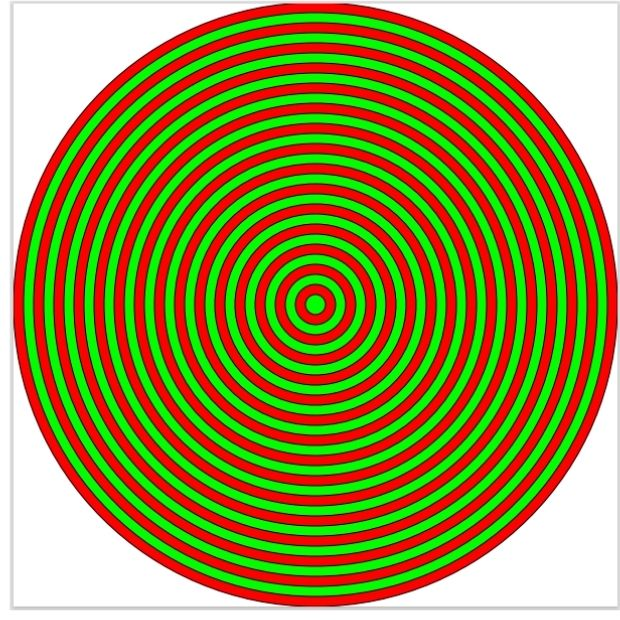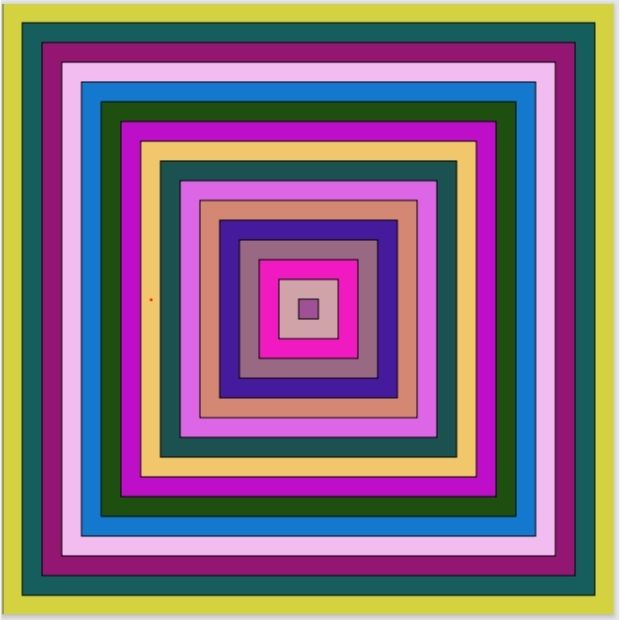# Using shapes like Circles & rectangles to PDF file

PDF

PDF pages in Python to add shapes like circles with random colour fills using ReportLab drawing.

Circles can be drawn by specifying centre X , Y coordinates and by giving radios. We can also specify the colour to be used to fill the circle. Here two circles are drawn with two different background-colors.

## With two alternate coloured circles``````from reportlab.graphics.shapes import *
my_path='G:\\My drive\\testing\\pypdf2\\my_pdf.pdf'
d=Drawing(600,600)
import random
for rd in range(0,300,10):
if(rd%20==0):
else:
from reportlab.graphics import renderPDF
renderPDF.drawToFile(d,my_path,'')``````

## Raondom Multi coloured concentric circles

We can generate float between 0 and less than 1 ( exclusive ) by using random.random().

random.uniform() returns between start and stop ( inclusive )``````from reportlab.graphics.shapes import *
my_path='G:\\My drive\\testing\\pypdf2\\my_pdf.pdf'
d=Drawing(1200,1200)
import random
for rd in range(0,600,20):
r=random.random()
g=random.random()
b=random.random()

from reportlab.graphics import renderPDF
renderPDF.drawToFile(d,my_path,'')``````

## Drawing random colour filled rectanglesHere we have used random.uniform() to generate random floats between 0 and 1 (inclusive of 0 and 1). Check this link for difference between random.uniform() and random.random()

Here width and height is set to to variables. So it can easily increased or decresed at single point.
``w,h=620,620``
Full code is here.
``````from reportlab.graphics.shapes import *
my_path='G:\\My drive\\testing\\pypdf2\\my_pdf.pdf'
w,h=620,620
d = Drawing(w,h)
import random
for rd in range(0,int(w/2),20):
r=random.uniform(0,1)
g=random.uniform(0,1)
b=random.uniform(0,1)
d.add(Rect(rd, rd, w-(rd*2), h-(rd*2), fillColor=[r,g,b]))

from reportlab.graphics import renderPDF
renderPDF.drawToFile(d, my_path, '')``````

Subscribe to our YouTube Channel here

## Subscribe

* indicates required
Subscribe to plus2netplus2net.com

Post your comments , suggestion , error , requirements etc here